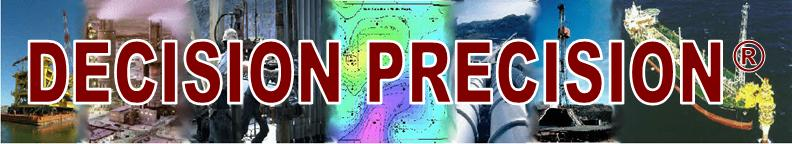"A Good Science and Engineering Decision is a Good Business Decision"

Decision Precision training and consulting Decision Analysis Levels of Proficiency

The days of training required depends greatly upon the experience and capabilities of the participants. Here are four proficiency levels and approximate days of training:

## AWARENESS 1 to 4 hours

• Ten-step decision analysis process
• Understanding that cash flow drives business value
• Using present value discounting for time value of money
• Expected value concept and solving simple decision trees
• Expected Monetary Value decision rule

Note: Executive Workshops range from 1 hour to 1 day in length. Depending upon the interests of the participants, the discussion ranges from how to interpret and use the results of a decision analysis and the calculation fundamentals -to- risk policy and measuring shareholder value creation.

## WORKING KNOWLEDGE 0.5-3 days

• Decision policy; why risk aversion usually can be neglected
• Traditional decision criteria and strengths/weaknesses
• Complement, addition and multiplication rules
• Probability distributions; mean and standard deviation
• How Monte Carlo simulation works
• Simple spreadsheet modeling and using a decision analysis tool (Crystal Ball, @RISK, DATA, PrecisionTree or other decision analysis software)
• Designing decision models for Monte Carlo simulation and decision tree analysis
• Multi-discipline team analyses

## COMPETENCE 2 to 7 days

• Modeling inflation and escalation
• Bayes Theorem
• Value of information problems
• Proficient in spreadsheet modeling: layout, names, functions, debugging, documenting
• Competence in a decision analysis computer tool (see above)
• Modeling dependencies; representing correlation
• Diagramming techniques (e.g., influence, tornado, sensitivity and spider diagrams; thought maps)
• Judgments and biases; feedback; post-audits
• Cost of capital; capital constraints; simple optimization methods
• Project schedule models and project risk management

## PROFICIENCY experience with substantial real-world problems

• Experience in constructing medium-sized decision models (over 50 variables)
• Portfolio modeling and optimization
• Optimizer's curse and winner's curse phenomena
• Competitive bidding
• Portfolio theory; CAPM alternative
• Risk policy calculations using a utility function
• Traditional optimization techniques: LP and mathematical programming
• Emerging technologies: simulated annealing, expert systems, fuzzy logic, chaos theory, analytic hierarchy process, multi-criteria decision-making
• Evaluation project control, review and documentation
• Analysis write-up and presentation